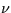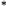Next: 13 Assessing NEWSIPS Data Up: 12 Final Archive Data Previous: 12.8 Low-Dispersion Merged Extracted

12.9 High-Dispersion Merged Extracted Image FITS File (MXHI)

The wavelengths,flags, and fluxes extracted from the SIHI are stored in the MXHI as a binary table extension using fixed-length floating point vectors. No primary data or additional extensions are included.

The binary table contains 17 fields of various data types. All vectors are padded with zeroes (both before and after the extracted data) to maintain a fixed length of 768 points. Wavelengths are uniformly sampled for each order, are measured in vacuum, and have had the heliocentric velocity correction applied. The width of each row (i.e., 65 + 22 × 768 = 16961) bytes, and the number of rows (i.e., NAXIS2) is equal to the number of extracted orders. In this manner, all the information pertaining to one spectral order is contained in one row of the binary table. The fields are defined in the order shown below:

• Order number, one 8-bit byte.
• Number of extracted points n, one 16-bit integer.
• Starting wavelength, one double-precision floating point value.
• Starting pixel at starting wavelength, one 16-bit integer.
• Wavelength increment, one double-precision floating point value.
• Slit height in pixels, one single-precision floating point number.
• Line number for found centroid of spectrum, one single-precision floating point number.
• Net flux spectrum, 768 single-precision floating point numbers with n extracted data points.
• Background flux spectrum, 768 single-precision floating point numbers with n extracted data points.
• Noise vector, 768 single-precision floating point numbers with n extracted data points.
•flags as n 16-bit integers stored in two's complement form.
• Ripple-corrected net flux spectrum, 768 single-precision floating with n extracted data points.
• Absolutely-calibrated, ripple-corrected net flux spectrum, 768 single-precision floating point numbers. with n extracted data points.
• Start pixel for background fit, one 16-bit integer number. *
• End pixel for background fit, one 16-bit integer number. *
• Chebyshev scale factor, one single-precision floating point number. *
• Chebyshev polynomial coefficients for global background correction, 7 single-precision floating point numbers. *
Note that unlike the MXLO, SILO, and SIHI, the starting wavelengths listed in the MXHI table do not refer to the first data point in the flux vectors, but rather the starting pixel listed in field four. In this manner, the 768-point flux vector can be mapped directly to the 768-pixel wide high-dispersion SI array.

As in low dispersion, since the absolute calibration covers the range of 1150-1980Å for short-wavelength spectra and 1850-3350Å for long-wavelength spectra, data points outside this wavelength range are set to 0 in the absolutely-calibrated flux vector. The net, background, and noise vectors are not affected. (Note that unlike the sigma vector in the MXLO file, the MXHI noise vector is uncalibrated.) Uncalibrated data points are also flagged in theflag vector with a value of -2. Table 12.11 shows the basic FITS Keywords for the MXHI.IMPORTANT NOTE: Several adjustments must be made to the last four parameters (fields 14-17) if the user wishes to evaluate the Chebyshev coefficients in order to reproduce the background fluxes as stored in the ninth field of the MXHI extension header. First, the parameters have inadvertently been stored in the reverse order (i.e., the parameters written in the first row of the table should have been stored in the last row, the parameters for the second row in the second to last row, etc.). So, for example, in the case of the LWR camera, the starting and ending pixels, Chebyshev scale factor, and Chebyshev coefficients found in row 1 (echelle order 127) actually pertain to row 61 (echelle order 67). Second, the true starting pixel is 768 minus the stored ending pixel and the true ending pixel is 768 minus the stored starting pixel. These true pixel values must be used to correctly evaluate the Chebyshev coefficients. Third, once the Chebyshev coefficients have been evaluated, the resultant background fluxes'' must be scaled in the following manner: multiply each background value by both the Chebyshev scale factor and the corresponding extraction slit height then divide this result by 32. Finally, the resultant array of background fluxes which are produced upon evaluation of the Chebyshev coefficients must be reversed (i.e., the computed background flux for pixel 1 becomes the background flux for pixel 768 and vice versa). We emphasize that these reversals and scalings are needed only when using the Chebyshev parameters in fields 14-17 to reproduce the background fluxes-the background fluxes themselves as contained in the ninth field are correct.

Table 12.11:  MXHI - Basic FITS Keywords
Keyword and value Description
 SIMPLE = T Standard FITS Format
 BITPIX = 8 Binary data
 NAXIS = 0 No image data
 EXTEND = T Extensions are present
 TELESCOP= 'IUE '  International Ultraviolet Explorer
 DATE = 'dd/mm/yy'  Date file was written
 ORIGIN = 'VILSPA '  Institution generating the file
 XTENSION= 'BINTABLE'  Binary table extension
 BITPIX = 8 Binary data
 NAXIS = 2 Two-dimensional table array
 NAXIS1 = 16961 Width of row in bytes
 NAXIS2 = nn Number of orders
 PCOUNT = 0 Number of bytes following data matrix
 GCOUNT = 1 Only one group
 TFIELDS = 17 Number of columns in the table
 TFORM1 = '1B '  8-bit byte
 TTYPE1 = 'ORDER '  Order number
 TUNIT1 = ' '  Unitless
 TFORM2 = '1I '  16-bit integer
 TTYPE2 = 'NPOINTS '  Number of non-zero points
 TUNIT2 = ' '  Unitless
 TFORM3 = '1D '  Double precision
 TTYPE3 = 'WAVELENGTH'  Starting wavelength
 TUNIT3 = 'ANGSTROM'  Unit is Angstrom
 TFORM4 = '1I '  16-bit integer
 TTYPE4 = 'STARTPIX'  Starting pixel at starting wavelength
 TUNIT4 = 'PIXEL '  Unit is pixel
 TFORM5 = '1D '  Double precision value
 TTYPE5 = 'DELTAW '  Wavelength increment
 TUNIT5 = 'ANGSTROM'  Unit is Angstrom
 TFORM6 = '1E '  Single precision
 TTYPE6 = 'SLIT HEIGHT'  Height of extraction slit
 TUNIT6 = 'PIXEL '  Unit is pixel
 TFORM7 = '1E '  Single precision
 TTYPE7 = 'LINE_FOUND'  Line number where spectral centroid is found
 TUNIT7 = 'PIXEL '  Unit is pixel
 TFORM8 = '768E '  Single precision array
 TTYPE8 = 'NET '  Net flux array
 TUNIT8 = 'FN '  Unit is IUE Flux Number (FN)
 TFORM9 = '768E '  Single precision array
 TTYPE9 = 'BACKGROUND'  Background flux array
 TUNIT9 = 'FN '  Unit is IUE Flux Number(FN)
 TFORM10 = '768E '  Single precision array
 TTYPE10 = 'NOISE '  Noise spectrum
 TUNIT10 = 'FN '  Unit is IUE Flux Number (FN)
 TFORM11 = '768I '  16-bit integer array
 TTYPE11 = 'QUALITY '  Data quality flag
 TUNIT11 = ' '  Unitless
 TFORM12 = '768E '  Single precision array
 TTYPE12 = 'RIPPLE '  Ripple-corrected net flux array
 TUNIT12 = 'FN '  Unit is IUE Flux Number (FN)
 TFORM13 = '768E '  Single precision array
 TTYPE13 = 'ABS_CAL '  Absolutely-calibrated net flux
 TUNIT13 = 'ERGS/CM2/S/A'  Unit is ergs/cm2/sec/Angstrom
 TFORM14 = '1I '  16-bit integer
 TTYPE14 = 'START-BKG'  Beginning pixel of background fit
 TUNIT14 = 'PIXEL '  X-axis in SIHI image
 TFORM15 = '1I '  16-bit integer
 TTYPE15 = 'END-BKG '  End pixel of background fit
 TUNIT15 = 'PIXEL '  X-axis in SIHI image
 TFORM16 = '1E '  Single precision
 TTYPE16 = 'SCALE_BKG'  Chebychev scale factor
 TUNIT16 = ' '  Unitless
 TFORM17 = '7E '  Single precision array
 TTYPE17 = 'COEFF '  Chebychev coefficients of background fit
 TUNIT17 = ' '  Unitless
 FILENAME= 'AAAnnnnn.MXHI'  Filename (camera) (number) .MXHI
 EXTNAME = 'MEHI '  Name of tableNext: 13 Assessing NEWSIPS Data Up: 12 Final Archive Data Previous: 12.8 Low-Dispersion Merged Extracted
Karen Levay
12/4/1997